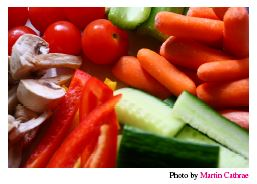Competitions

# Boiling VegetablesThe trick to boiling vegetables is to make sure all pieces are about the same size. If they are not, the small ones get too soft or the large ones get undercooked (or both). Fortunately, you have heard of the kitchen knife, but your parents' warnings of using sharp instruments still echoes in your head. Therefore you better use it as little as possible. You can take a piece of a vegetable of weight w and cut it arbitrarily in two pieces of weight wleft and wright, where wleft + wright = w. This operation constitutes a "cut".

Given a set of pieces of vegetables, determine the minimum number of cuts needed to make the ratio between the smallest and the largest resulting piece go above a given threshold.

#### Input

Starts with a floating point number t (0.5 < t < 1) with 2 decimal digits, and a positive integer n (n1000). Next follow n positive integer weights w1, w2, ..., wn. All weights are less than 106.

#### Output

Output the minimum number of cuts needed to make the ratio between the resulting minimum weight piece and the resulting maximum weight piece be above t. You may assume that the number of cuts needed is less than 500. To avoid issues with floating point numbers, you can assume that the optimal answer for ratio t is the same as for ratio t + 0.0001.

Time limit 1 second
Memory limit 128 MiB
Input example #1
0.99 3
2000 3000 4000

Output example #1
6

Input example #2
0.80 2
1000 1400

Output example #2
3

Source 2013 ACM Nordic (NCPC), October 5, Problem B